## Example Questions

### Example Question #1 : Proportion / Ratio / Rate

A solution made up ofalcohol by volume is mixed withliters of solution that isalcohol by volume. How much, in liters, of thealcoholic solution is needed to make a mixture that isalcohol by volume?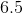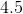Explanation:

Letrepresent the number of liters of the 40% solution. Then it follows thatliters of the 40% solution plus 4 liters of the 10% solution will equal (x+4) liters of a 25% solution. This can be represented by the following equation: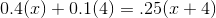Now solve for x: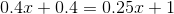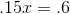You will need 4 liters of the 40% solution in order to make a mixture that is 25% alcohol by volume

### Example Question #2 : Proportion / Ratio / Rate

A solution isparts water,parts wine, andpart honey.  If a container of this solution containsgallons of water, how much total solution is there in it?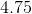gallons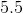gallonsgallonsgallonsgallonsgallons

Explanation:

To begin, notice that there is a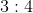ratio between the water in your container and the water specified by the mix of the components.  Given that there aretotal parts in your solution, this means that you can set up this equation: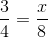Multiplying both sides by 8, you get: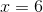There aretotal gallons of solution.

### Example Question #3 : Proportion / Ratio / Rate

A solution is made up ofparts water,parts orange juice, andparts apple juice.  If you wanted the percentage of orange juice to be% of the solution, how many parts would you need to add?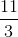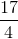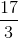Explanation:

To begin, you know that the basic form of the solution has a total of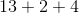, orparts.  Now, we know that we are going to have to addparts of orange juice.  This means that the new solution will have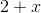parts orange juice and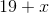total parts (since we are adding to the original).  Since we want this to be% orange juice, we really want the following equation to be true: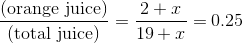Solve the following equation, therefore: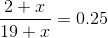Multipy by: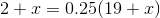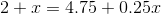Now, isolate: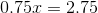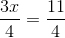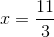Tired of practice problems?

Try live online GRE prep today.